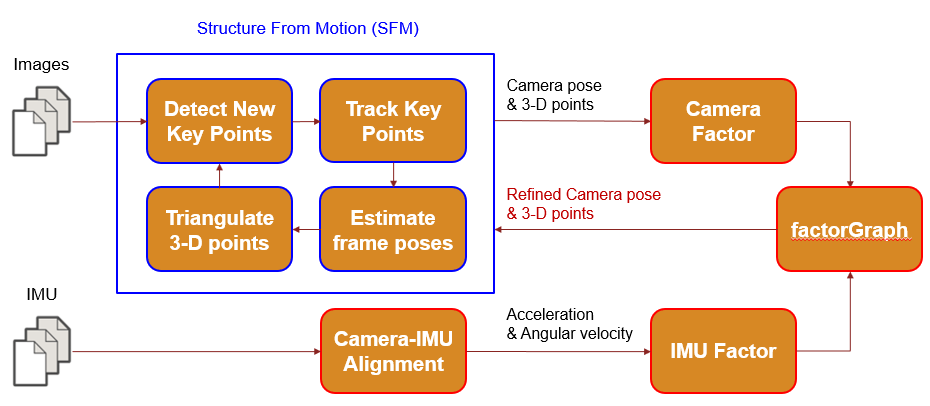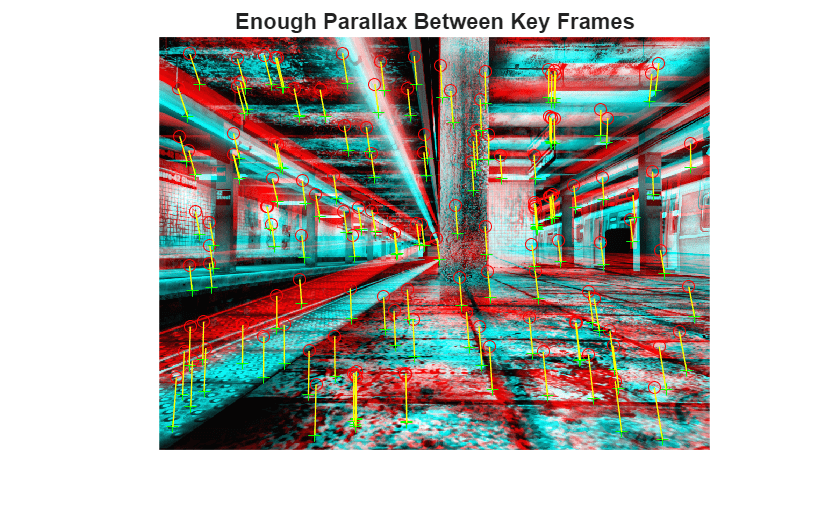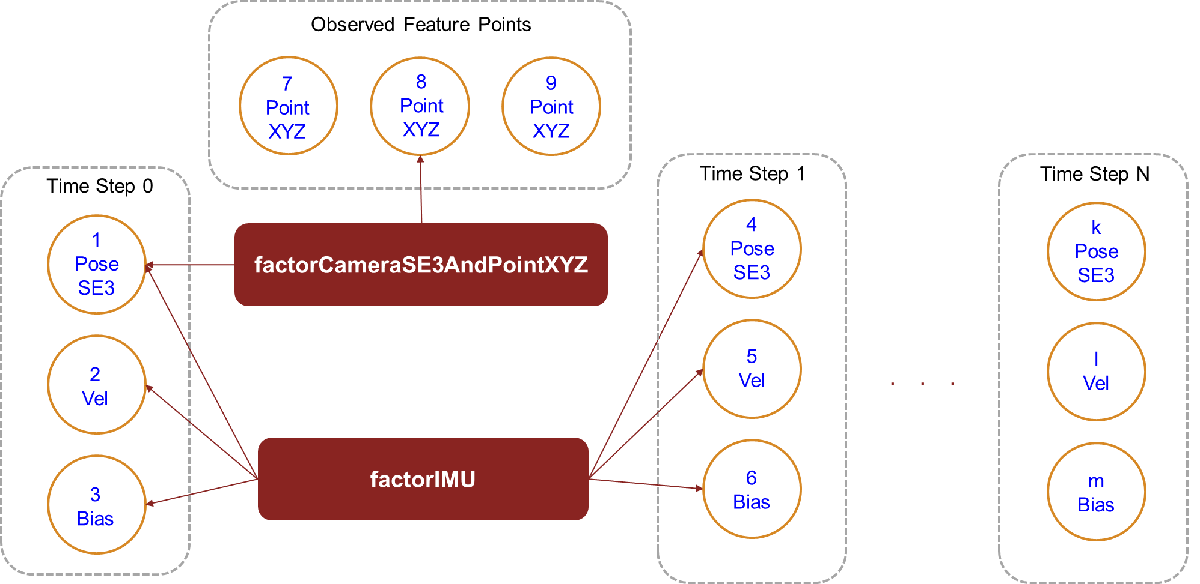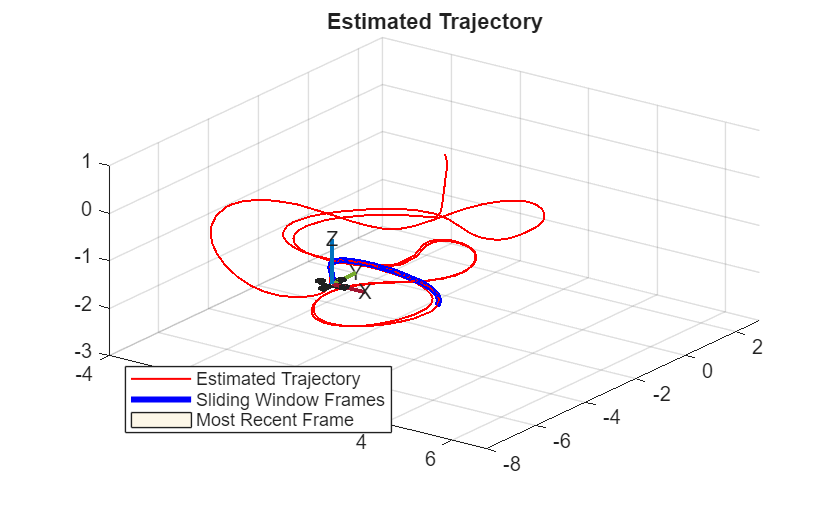# Monocular Visual-Inertial Odometry Using Factor Graph

Monocular visual-inertial odometry estimates the position and orientation of the robot using camera and inertial measurement unit (IMU) sensor data. Camera-based state estimation is accurate during low-speed navigation. However, camera-based estimation faces challenges such as motion blur and track loss at higher speeds. Also monocular camera-based estimation can estimate poses at an arbitrary scale. On the other hand, inertial navigation can handle high-speed navigation easily and estimate poses at world scale. You can combine the advantages of both types of sensor data to achieve better accuracy using tightly coupled factor graph optimization.### Overview

The visual-inertial system implemented in this example consists of a simplified version of the monocular visual odometry front-end of the VINS  algorithm and a factor graph back-end.

The visual odometry front-end has responsibilities similar to standard structure from motion (SfM) algorithms, such as oriented FAST and rotated BRIEF (ORB) and simultaneous localization and mapping (SLAM). The visual odometry front-end detects and tracks key points across multiple frames, estimates camera poses, and triangulates 3-D points using multi-view geometry. The factor graph back-end jointly optimizes the estimated camera poses, 3-D points, IMU velocity, and bias. Before fusing the camera and IMU measurements, you must align the camera and IMU to compute the camera pose scale, gravity rotation, and initial IMU velocity and bias.### Set Up

This example uses the Blackbird data set (NYC Subway Winter) to demonstrate the visual-inertial odometry workflow. Download the data set.

`data = helperDownloadData();`

Fix the random seed for repeatability.

`rng(0)`

#### Initialize Algorithm Parameters

Use the `helperVIOParameters` function to initialize and tune these parameters:

#### Visual Front-End Parameters

• Random sample consensus (RANSAC) threshold (`F_Threshold`), confidence (`F_Confidence)`, and iterations (`F_Iterations)`

• Kanade-Lucas-Tomasi (KLT) tracker bidirectional error (`KLT_BiErr`), number of levels (`KLT_Levels`), and block size (`KLT_Block`)

• Minimum parallax for key frame selection and triangulating new 3-D points

• Minimum number of key points to track in each frame (`numTrackedThresh`)

• Maximum number of key points to track in each frame (`maxPointsToTrack`)

#### Factor Graph Optimization Back-End Parameters

• Factor graph solver options (`SolverOpts`)

• Sliding window size (`windowSize`) - Maximum number of frames in the sliding window.

• Frame rate at which to run the sliding window optimization (`optimizationFrequency`)

```params = helperVIOParameters(); % Set to true if IMU data is available. useIMU = true;```

Initialize variables.

```status = struct("firstFrame",true,"isMapInitialized",false,"isIMUAligned",false,"Mediandepth",false); % Set to true to attempt camera-IMU alignment. readyToAlignCameraAndIMU = false; % Set initial scene median depth. initialSceneMedianDepth = 4; viewId = 0; removedFrameIds = []; allCameraTracks = cell(1,5000); % Enable visualization. vis = true; showMatches = false; if vis % Figure variables. axMatches = []; axTraj = []; axMap = []; end```

Set up factor graph for back-end tightly coupled factor graph optimization.

```% Set up factor graph for fusion. slidingWindowFactorGraph = factorGraph; maxFrames = 10000; maxLandmarks = 100000; ids = helperGenerateNodeID(slidingWindowFactorGraph,maxFrames,maxLandmarks); % Information matrix (measure of accuracy) associated with the camera % projection factors that relate 3-D points and estimated camera poses. cameraInformation = ((data.intrinsics.K(1,1)/1.5)^2)*eye(2); % Initialize IMU parameters. The fusion accuracy depends on these parameters. imuParams = factorIMUParameters(SampleRate=100,GyroscopeNoise=0.1, ... GyroscopeBiasNoise=3e-3,AccelerometerNoise=0.3, ... AccelerometerBiasNoise=1e-3,ReferenceFrame="ENU");```

Create the point tracker to track key points across multiple frames.

```tracker = vision.PointTracker(MaxBidirectionalError=params.KLT_BiErr, ... NumPyramidLevels=params.KLT_Levels,BlockSize=params.KLT_Block);```

Set up the feature manager to maintain key point tracks.

```fManager = helperFeaturePointManager(data.intrinsics,params,maxFrames,maxLandmarks); % Set up the key point detector. fManager.DetectorFunc = @(I)helperDetectKeyPoints(I);```

Create an image view set to maintain frame poses.

`vSet = imageviewset;`

Specify the first and last frames to process from the data set. Then, process the first frame.

```% IMU data is available from frame number 40 in the data set. startFrameIdx = 40; % Index of the last frame to process in this example. For reasonable % example execution time, process up to only frame 1000 of the data set. endFrameIdx = 1000; allFrameIds = startFrameIdx:endFrameIdx; % In the first frame, detect new key points and initialize the tracker for % future tracking. status.firstFrame = false; I = data.images{startFrameIdx}; if params.Equalize % Enhance contrast if images are dark. I = adapthisteq(I,NumTiles=params.NumTiles,ClipLimit=params.ClipLimit); end if params.Undistort % Undistort if images contain perspective distortion. I = undistortImage(I,data.intrinsics); end % Assign a unique view ID for each processed camera frame or image. viewId = viewId + 1; currPoints = createNewFeaturePoints(fManager,I); updateSlidingWindow(fManager,I,currPoints,true(size(currPoints,1),1),viewId); initialize(tracker, currPoints, I); prevI = I; firstI = I; vSet = addView(vSet,viewId,rigidtform3d);```

Begin a loop through the entire dataset.

`for curIdx = allFrameIds(2:end)`

### Image Preprocessing

Image preprocessing involves these steps:

• Equalize — Enhance the contrast of an image to correct for dim lighting, which can affect feature extraction and tracking.

• Undistort — Correct for radial and tangential distortions that can impact state estimation.

``` % Read image data. I = data.images{curIdx}; if params.Equalize % Enhance contrast if images are dark. I = adapthisteq(I,NumTiles=params.NumTiles,ClipLimit=params.ClipLimit); end if params.Undistort % Undistort if images contain perspective distortion. I = undistortImage(I,data.intrinsics); end % Assign a unique view ID for each processed camera frame or image. viewId = viewId + 1;```

### Feature Tracking

To compute a camera frame pose, you must calculate 2D-2D correspondences (2-D image point tracks across multiple frames). There are several ways to estimate 2-D feature points that see the same landmark (key point tracks), but this example uses a Kalman tracker for tracking feature points in multiple images.

Tracks are not all accurate and can contain outliers. Tracking performance also depends on the Kalman tracker parameters, such as bidirectional error. Even in an ideal case, you can expect some invalid tracks, such as those due to repetitive structures. As such, outlier rejection is a critical task in feature tracking. To reject outliers from tracks, use fundamental matrix decomposition in the feature point manager while updating the sliding window with the latest feature point tracks.

``` % Track previous frame points in the current frame. [currPoints,validIdx] = tracker(I); if status.isMapInitialized [prevPoints,pointIds,isTriangulated] = getKeyPointsInView(fManager,viewId-1); end % Update the sliding window after tracking features in the current % frame. If the sliding window already contains maximum number of % frames specified using windowSize, one frame with id % removeFrameId will be removed from the window to accommodate % space for the current frame. [removedFrameId,windowState] = updateSlidingWindow(fManager,I,currPoints,validIdx,viewId); if (removedFrameId > fManager.slidingWindowViewIds(1)) % Store non-key frames or removed frame IDs. removedFrameIds(end + 1) = removedFrameId; %#ok end ```

Visualize the feature point tracks between the last key frame and current frame.

``` if status.isMapInitialized svIds = getSlidingWindowIds(fManager); if length(svIds) > 2 [matches1,matches2] = get2DCorrespondensesBetweenViews(fManager,svIds(end-2),viewId); if vis && showMatches if isempty(axMatches) axMatches = axes(figure); %#ok end % Visualize matches between the last key frame and the % current view. showMatchedFeatures(data.images{allFrameIds(svIds(end-2))},I,matches1,matches2, ... Parent=axMatches); end end end```

### Initial Structure from Motion (SfM)

The accelerometer and gyroscope readings of the IMU data contain some bias and noise. To estimate bias values, you must obtain accurate pose estimates between the first few frames. You can achieve this by using SfM. SfM involves these major steps:

• When there is enough parallax between the first key frame and the current frame, estimate the relative pose between the two, using 2D-2D correspondences (key point tracks across multiple frames).

• Triangulate 3-D points using the world poses of key frames and 2D-2D correspondences.

• Track the 3-D points in the current frame, and compute the current frame pose using 3D-2D correspondences.

``` if ~status.isMapInitialized if windowState.FirstFewViews % Accept the first few camera views. vSet = addView(vSet,viewId,rigidtform3d); elseif windowState.EnoughParallax % Estimate relative pose between the first key frame in the % window and the current frame. svIds = getSlidingWindowIds(fManager); [matches1,matches2] = get2DCorrespondensesBetweenViews(fManager,svIds(end-1),svIds(end-0)); valRel = false(size(matches1,1),1); for k = 1:10 [F1,valRel1] = estimateFundamentalMatrix( ... matches1,matches2,Method="RANSAC", ... NumTrials=params.F_Iterations,DistanceThreshold=params.F_Threshold, ... Confidence=params.F_Confidence); if length(find(valRel)) < length(find(valRel1)) valRel = valRel1; F = F1; end end inlierPrePoints = matches1(valRel,:); inlierCurrPoints = matches2(valRel,:); relPose = estrelpose(F,data.intrinsics, ... inlierPrePoints,inlierCurrPoints); % Get the table containing the previous camera pose. prevPose = rigidtform3d; % Compute the current camera pose in the global coordinate % system relative to the first view. currPose = relPose; %vSet = addView(vSet,svIds(end-1),currPose); vSet = addView(vSet,viewId,currPose); status.isMapInitialized = true; axisSFM = axes(figure); %#ok showMatchedFeatures(firstI,I,matches1,matches2, ... Parent=axisSFM); title(axisSFM,"Enough Parallax Between Key Frames"); end else```### Camera-IMU Alignment

To optimize camera and IMU measurements, you must align them by bringing them to the same base coordinate frame and scale. Alignment primarily consists of these major tasks:

• Compute the camera pose scale to make it similar to the IMU or world scale.

• Calculate the gravity rotation required to rotate gravity vector from local navigation reference frame of IMU to initial camera reference frame. The inverse of this rotation aligns the z-axis of the camera with the local navigation reference frame.

• Estimate the initial IMU bias.

``` if ~status.isIMUAligned && readyToAlignCameraAndIMU svIds = getSlidingWindowIds(fManager); % Because you have not yet computed the latest frame pose, % So use only the past few frames for alignment. svIds = svIds(1:end-1); [gyro,accel] = helperExtractIMUDataBetweenViews( ... data.gyroReadings,data.accelReadings,data.timeStamps,allFrameIds(svIds)); [xyz] = getXYZPoints(fManager,xyzIds); % Align camera with IMU. camPoses = poses(vSet,svIds); [gRot,scale,info] = ... estimateGravityRotationAndPoseScale(camPoses,gyro,accel, ... SensorTransform=data.camToIMUTransform,IMUParameters=imuParams); disp("Estimated scale: " + scale);```

If the alignment is successful, update the camera poses, 3-D points, and add IMU factors between the initial frames in the current sliding window.

``` if info.IsSolutionUsable && scale > 1e-3 status.isIMUAligned = true; posesUpdated = poses(vSet); % Transform camera poses to navigation frame using % computed gravity rotation and pose scale. [posesUpdated,xyz] = helperTransformToNavigationFrame(posesUpdated,xyz,gRot,scale); vSet = updateView(vSet,posesUpdated); % Plot the scaled and unscaled estimated trajectory against % ground truth. if vis p1 = data.camToIMUTransform.transform(vertcat(camPoses.AbsolutePose.Translation)); axAlign = axes(figure); %#ok g1 = data.gTruth(allFrameIds(camPoses.ViewId),1:3); plot3(g1(:,1),g1(:,2),g1(:,3),"g",Parent=axAlign); hold(axAlign,"on") plot3(scale*p1(:,1),scale*p1(:,2),scale*p1(:,3),"r",Parent=axAlign); plot3(p1(:,1),p1(:,2),p1(:,3),"b",Parent=axAlign); hold(axAlign,"off") legend(axAlign,"Ground Truth","Estimated scaled trajectory","Estimated trajectory") title("Camera-IMU Alignment") drawnow end if status.isIMUAligned % After alignment, add IMU factors to factor graph. for k = 1:length(gyro) nId = [ids.pose(svIds(k)),ids.vel(svIds(k)),ids.bias(svIds(k)), ... ids.pose(svIds(k+1)),ids.vel(svIds(k+1)),ids.bias(svIds(k+1))]; fIMU = factorIMU(nId,gyro{k},accel{k},imuParams,SensorTransform=data.camToIMUTransform); slidingWindowFactorGraph.addFactor(fIMU); end end % Set camera pose node guesses and 3-D point guesses % after alignment. slidingWindowFactorGraph.nodeState( ... ids.pose(svIds), ... helperCameraPoseTableToSE3Vector( ... poses(vSet,svIds))); slidingWindowFactorGraph.nodeState( ... ids.point3(xyzIds),xyz);```

Estimate an initial guess for IMU bias by using factor graph optimization with the camera projection and IMU factors.

``` % Add prior to first camera pose to fix it softly during % optimization. fixNode(slidingWindowFactorGraph,ids.pose(svIds)); fixNode(slidingWindowFactorGraph,ids.point3(xyzIds)); % Add velocity prior to first IMU velocity node. fVelPrior = factorVelocity3Prior(ids.vel(svIds(1))); addFactor(slidingWindowFactorGraph,fVelPrior); % Add bias prior to first bias node. fBiasPrior = factorIMUBiasPrior(ids.bias(svIds(1))); addFactor(slidingWindowFactorGraph,fBiasPrior); % Perform visual-inertial optimization after alignment to estimate % initial IMU bias values. soll1 = optimize(slidingWindowFactorGraph, ... params.SolverOpts); fixNode(slidingWindowFactorGraph,ids.pose(svIds),false); fixNode(slidingWindowFactorGraph,ids.point3(xyzIds),false); fixNode(slidingWindowFactorGraph,ids.pose(svIds(1))); soll = optimize(slidingWindowFactorGraph, ... params.SolverOpts); fixNode(slidingWindowFactorGraph,ids.pose(svIds(1)),false); % Update feature manager and view set after optimization. vSet = updateView(vSet,helperUpdateCameraPoseTable(poses(vSet,svIds), ... slidingWindowFactorGraph.nodeState( ... ids.pose(svIds)))); xyz = slidingWindowFactorGraph.nodeState( ... ids.point3(xyzIds)); setXYZPoints(fManager,xyz,xyzIds); end end```
```Estimated scale: 1.9661 ```### IMU Pose Prediction

When IMU data is available, you can predict the world pose of the camera by integrating accelerometer and gyroscope readings. Use factor graph optimization to further refine this prediction.

``` imuGuess = false; if status.isIMUAligned % Extract gyro and accel reading between current image frame % and last acquired image frame to create IMU factor. svIds = getSlidingWindowIds(fManager); svs = svIds((end-1):end); [gyro,accel] = helperExtractIMUDataBetweenViews(data.gyroReadings, ... data.accelReadings,data.timeStamps,allFrameIds(svs)); nodeID = [ids.pose(svs(1)) ... ids.vel(svs(1)) ... ids.bias(svs(1)) ... ids.pose(svs(2)) ... ids.vel(svs(2)) ... ids.bias(svs(2))]; % Create the transformation required to trasform a camera pose % to IMU base frame for the IMU residual computation. fIMU = factorIMU(nodeID,gyro{1},accel{1},imuParams, ... SensorTransform=data.camToIMUTransform); % Add camera pose and IMU factor to graph. slidingWindowFactorGraph.addFactor(fIMU); % Set velocity and bias guess. prevP = nodeState(slidingWindowFactorGraph,ids.pose(svs(1))); prevVel = nodeState(slidingWindowFactorGraph,ids.vel(svs(1))); prevBias = nodeState(slidingWindowFactorGraph,ids.bias(svs(1))); [pp,pv] = fIMU.predict(prevP,prevVel,prevBias); imuGuess = true; end [currPoints,pointIds,isTriangulated] = getKeyPointsInView(fManager,viewId); cVal = true(size(currPoints,1),1); cTrf = find(isTriangulated);```

If no IMU prediction is available, then use 3D-2D correspondences to estimate the current view pose.

``` if ~imuGuess x3D = getXYZPoints(fManager,pointIds(isTriangulated)); c2D = currPoints(isTriangulated,:); ii = false(size(x3D,1),1); currPose = rigidtform3d; for k = 1:params.F_loop [currPosel,iil] = estworldpose( ... currPoints(isTriangulated,:),x3D, ... data.intrinsics,MaxReprojectionError=params.F_Threshold,Confidence=params.F_Confidence, ... MaxNumTrials=params.F_Iterations); if length(find(ii)) < length(find(iil)) ii = iil; currPose = currPosel; end end cVal(cTrf(~ii)) = false; else```

Use the IMU predicted pose as an initial guess for motion-only bundle adjustment.

``` x3D = getXYZPoints(fManager,pointIds(isTriangulated)); c2D = currPoints(isTriangulated,:); [currPose,velRefined,biasRefined,ii] = helperBundleAdjustmentMotion( ... x3D,c2D,data.intrinsics,size(I),pp,pv,prevP,prevVel,prevBias,fIMU); slidingWindowFactorGraph.nodeState( ... ids.vel(viewId),velRefined); slidingWindowFactorGraph.nodeState( ... ids.bias(viewId),biasRefined); cVal(cTrf(~ii)) = false; end setKeyPointValidityInView(fManager,viewId,cVal); vSet = addView(vSet,viewId,currPose);```

Add camera projection factors related to the 3-D point tracks of the current view.

``` obs2 = pointIds(isTriangulated); obs2 = obs2(ii); fCam = factorCameraSE3AndPointXYZ( ... [ids.pose(viewId*ones(size(obs2))) ids.point3(obs2)], ... data.intrinsics.K,Measurement=c2D(ii,:), ... Information=cameraInformation); allCameraTracks{viewId} = [viewId*ones(size(obs2)) obs2 fCam.Measurement]; slidingWindowFactorGraph.addFactor(fCam); end```

### 3-D Point Triangulation

When using the latest 2D-2D correspondences for camera-world pose estimation, you must frequently create new 3-D points.

``` if status.isMapInitialized [newXYZ,newXYZID,newPointViews,newPointObs] = triangulateNew3DPoints(fManager,vSet); if isempty(axMap) && windowState.WindowFull axMap = axes(figure); %#ok % Plot the map created by Initial SfM helperPlotCameraPosesAndLandmarks(axMap,fManager,vSet,removedFrameIds,true); end```### Estimated Pose Refinement Using Factor Graph Optimization

Factor graph optimization reduces the error in trajectory or camera pose estimation. Various factors, like inaccurate tracking and outliers, can contribute to estimation errors.

Graph optimization adjusts camera poses to satisfy various sensor measurement constraints, like camera observations (3-D point projection onto an image-frame-generating 2-D image point observation), IMU relative poses, and relative velocity change. You can categorize optimization based on the type of factors used.

• Bundle adjustment — Uses only camera measurements. `factorCameraSE3AndPointXYZ` is useful for adding camera measurement constraints to the graph.

• Visual-inertial optimization — Along with camera measurements, add IMU measurements, like gyroscope and accelerometer readings, to the graph by using `factorIMU`.

The visual-inertial factor graph system consists of these processes:

• Estimate the camera pose nodes at different timestamps, and connect them to both the camera projection and the IMU factors.

• Connect the 3-D point landmark nodes to the camera projection factor.

• Connect the IMU velocity and bias nodes to only IMU factors.Update the sliding window with the latest 3-D points and camera view pose.

``` newPointsTriangulated = false; if ~isempty(newXYZ) newPointsTriangulated = true; % Store all new 3D-2D correspondenses. for pIte = 1:length(newPointViews) allCameraTracks{newPointViews(pIte)} = [allCameraTracks{newPointViews(pIte)}; newPointObs{pIte}]; end obs = vertcat(allCameraTracks{:}); % Create camera projection factors with the latest % 3-D point landmark observations in the current image. fCam = factorCameraSE3AndPointXYZ([ids.pose(obs(:,1)) ... ids.point3(obs(:,2))],data.intrinsics.K, ... Measurement=obs(:,3:4), ... Information=cameraInformation); addFactor(slidingWindowFactorGraph,fCam); end % Set current camera pose node state guess. svIdds = getSlidingWindowIds(fManager); slidingWindowFactorGraph.nodeState(ids.pose(svIdds), ... helperCameraPoseTableToSE3Vector(poses(vSet,svIdds)));```

Refine the estimated camera frame poses and 3-D points using factor graph optimization. The optimization is time consuming. So, the optimization is not run after estimating the pose of each frame. The frame frequency at which the optimization is run can be controlled using a parameter.

``` if helperDecideToRunGraphOptimization(curIdx,newPointsTriangulated,params) % Recreate sliding window factor graph with only the latest key % frames, for performance. [slidingWindowFactorGraph,xyzIds] = helperRecreateSlidingWindowFactorGraph( ... slidingWindowFactorGraph,fManager,allCameraTracks,data.intrinsics,cameraInformation, ... imuParams,data.gyroReadings,data.accelReadings,data.timeStamps,allFrameIds,ids,data.camToIMUTransform); % Add guess for newly triangulated 3-D point node states. xyz = getXYZPoints(fManager,xyzIds); slidingWindowFactorGraph.nodeState( ... ids.point3(xyzIds),xyz); % Fix a few nodes during graph optimization % to fix the camera pose scale. Unfix them after optimization. if windowState.WindowFull fixNode(slidingWindowFactorGraph, ... ids.pose(fManager.slidingWindowViewIds(1:11))); else fixNode(slidingWindowFactorGraph, ... ids.pose(fManager.slidingWindowViewIds(1))); end if status.isIMUAligned % Fix the first velocity and bias nodes in the sliding % window. fixNode(slidingWindowFactorGraph, ... ids.vel(fManager.slidingWindowViewIds(1))); fixNode(slidingWindowFactorGraph, ... ids.bias(fManager.slidingWindowViewIds(1))); end % Optimize the sliding window. optiInfo = optimize(slidingWindowFactorGraph,params.SolverOpts);```

Update the feature manager and view set with your optimization results.

``` slidingWindowViewIds = getSlidingWindowIds(fManager); if ~status.Mediandepth status.Mediandepth = true; xyz = slidingWindowFactorGraph.nodeState( ... ids.point3(xyzIds)); medianDepth = median(vecnorm(xyz.')); [posesUpdated,xyz] = helperTransformToNavigationFrame(helperUpdateCameraPoseTable(poses(vSet,slidingWindowViewIds), ... slidingWindowFactorGraph.nodeState(ids.pose(slidingWindowViewIds))), ... xyz,rigidtform3d,initialSceneMedianDepth/medianDepth); % Set current camera pose node state guess. slidingWindowFactorGraph.nodeState(ids.pose(slidingWindowViewIds), ... helperCameraPoseTableToSE3Vector(posesUpdated)); % Add guess for newly triangulated 3-D points node states. slidingWindowFactorGraph.nodeState( ... ids.point3(xyzIds),xyz); else posesUpdated = helperUpdateCameraPoseTable(poses(vSet,slidingWindowViewIds), ... slidingWindowFactorGraph.nodeState( ... ids.pose(slidingWindowViewIds))); xyz = slidingWindowFactorGraph.nodeState( ... ids.point3(xyzIds)); end % Update the view set after visual-inertial optimization. vSet = updateView(vSet,posesUpdated); setXYZPoints(fManager,xyz,xyzIds); end end```

Add a new feature point to the Kalman tracker, in case the number of points goes below the feature tracking threshold.

``` createNewFeaturePoints(fManager,I); currPoints = getKeyPointsInView(fManager,viewId); setPoints(tracker,currPoints); if ~status.isIMUAligned && useIMU && status.isMapInitialized && windowState.WindowFull % The sliding window is full and the camera and IMU are not yet aligned. readyToAlignCameraAndIMU = true; end prevPrevI = prevI; prevI = I;```

Visualize the estimated trajectory.

``` if status.isMapInitialized && (mod(curIdx,10)==0) if vis if isempty(axTraj) axTraj = helperCreateTrajectoryVisualization([-4 7 -8 3 -3 1]); end % Visualize the estimated trajectory. helperVisualizeTrajectory(axTraj,fManager,vSet,removedFrameIds); end end end```Sample image of the scene.Plot all key frame camera poses and 3-D points. Observe the landmarks on features such as the ceiling, floor, and pillars.

`helperPlotCameraPosesAndLandmarks(axMap,fManager,vSet,removedFrameIds);`### Compare Estimated Trajectory with Ground Truth

As a measure of accuracy, compute these metrics:

• Absolute trajectory error (ATE) - Root Mean Squared Error (RMSE) between computed camera locations and ground truth camera locations.

• Scale error - Percentage of how far the computed median scale is to original scale.

Plot the estimated trajectory against the ground truth.

```addedFrameIds = allFrameIds(vSet.Views.ViewId); axf = axes(figure); helperPlotAgainstGroundTruth(vSet,data.gTruth,data.camToIMUTransform, ... addedFrameIds,axf,removedFrameIds);```Evaluate the tracking accuracy, based on root mean square error (RMSE) and median scale error.

`helperComputeErrorAgainstGroundTruth(data.gTruth,vSet,allFrameIds,removedFrameIds,data.camToIMUTransform);`
``` "Absolute RMSE for key frame trajectory (m): " "0.20406" "Percentage of median scale error: " "2.3038" ```

### Supporting Functions

This section details the short helper functions included in this example. Larger helper functions have been included in separate files.

`helperFeaturePointManager` manages key point tracks.

`helperVIOParameters` initializes visual-inertial odometry algorithm tunable parameters.

`helperBundleAdjustmentMotion` refines pose of current frame using motion-only bundle adjustment.

`helperSelectNewKeyPointsUniformly` selects specified number of newly created key points a specified distance from tracked points in current key frame.

`helperRecreateSlidingWindowFactorGraph` recreates factor graph with key frame data within current sliding window.

`helperCreateTrajectoryVisualization` creates trajectory plot with highlighted sliding window.

`helperVisualizeTrajectory` updates trajectory plot with latest data stored in view set and feature manager.

`helperPlotAgainstGroundTruth` plots estimated trajectory and ground truth trajectory for visual comparison.

`helperGenerateNodeID `generates unique factor graph node IDs for fixed number of camera view poses, IMU velocities, IMU biases, and 3-D point nodes.

```function ids = helperGenerateNodeID(fg,maxFrames,maxLandmarks) % helperGenerateNodeID ids.pose = generateNodeID(fg,[maxFrames 1]); ids.vel = generateNodeID(fg,[maxFrames 1]); ids.bias = generateNodeID(fg,[maxFrames 1]); ids.point3 = generateNodeID(fg,[maxLandmarks 1]); end```

`helperCameraPoseTableToSE3Vector` converts pose table to N-by-7 SE(3) pose matrix.

```function cameraPoses = helperCameraPoseTableToSE3Vector(cameraPoseTable) % helperCameraPoseTableToSE3Vector converts camera pose table returned by % poses method of imageviewset to N-by-7 SE3 pose vector format. cameraPoses = [cat(1,cameraPoseTable.AbsolutePose.Translation) rotm2quat(cat(3,cameraPoseTable.AbsolutePose.R))]; end```

`helperUpdateCameraPoseTable` updates pose table with latest estimated N-by-7 SE(3) poses.

```function cameraPoseTableUpdated = helperUpdateCameraPoseTable(cameraPoseTable,cameraPoses) % helperUpdateCameraPoseTable updates camera pose table with specified % N-by-7 SE(3) camera poses. cameraPoseTableUpdated = cameraPoseTable; R = quat2rotm(cameraPoses(:,4:7)); for k = 1:size(cameraPoses,1) cameraPoseTableUpdated.AbsolutePose(k).Translation = cameraPoses(k,1:3); cameraPoseTableUpdated.AbsolutePose(k).R = R(:,:,k); end end```

`helperDetectKeyPoints` detects key points.

```function keyPoints = helperDetectKeyPoints(grayImage) %helperDetectKeyPoints % Detect multi-scale FAST corners. keyPoints = detectORBFeatures(grayImage,ScaleFactor=1.2,NumLevels=4); % Uncomment any of the following or try different detectors to tune % keyPoints = detectFASTFeatures(grayImage,MinQuality=0.0786); % keyPoints = detectMinEigenFeatures(grayImage,MinQuality=0.01,FilterSize=3); end```

`helperDecideToRunGraphOptimization` decides whether to run or skip graph optimization at current frame.

```function shouldOptimize = helperDecideToRunGraphOptimization(curIdx,newPointsTriangulated,params) % helperDecideToRunGraphOptimization % If the current frame belongs to the initial set of frames, then run graph % optimization every frame, because the initial SfM is still running. % Otherwise, after a number of frames specified by optimization frequency, % run graph optimization. Lower frequency can result in a more accurate % estimation, but can increase execution time. numberOfInitialFrames = 250; shouldOptimize = (curIdx < numberOfInitialFrames) || (mod(curIdx,params.optimizationFrequency) == 0) || newPointsTriangulated; end```

`helperTransformToNavigationFrame` transforms and scales input poses and XYZ 3-D points to local navigation reference frame of IMU using gravity rotation and pose scale.

```function [posesUpdated,xyzUpdated] = helperTransformToNavigationFrame(poses,xyz,gRot,poseScale) % helperTransformToNavigationFrame transforms and scales the input poses and XYZ points % using specified gravity rotation and pose scale. posesUpdated = poses; % Input gravity rotation transforms the gravity vector from local % navigation reference frame to initial camera pose reference frame. % The inverse of this transforms the poses from camera reference frame % to local navigation reference frame. Ai = gRot.A'; for k = 1:length(poses.AbsolutePose) T = Ai*poses.AbsolutePose(k).A; T(1:3,4) = poseScale*T(1:3,4); posesUpdated.AbsolutePose(k) = rigidtform3d(T); end % Transform points from initial camera pose reference frame to % local navigation reference frame of IMU. xyzUpdated = poseScale*gRot.transformPointsInverse(xyz); end```

`helperExtractIMUDataBetweenViews` extracts IMU data between specified views.

```function [gyro,accel] = helperExtractIMUDataBetweenViews(gyroReadings,accelReadings,timeStamps,frameIds) % helperExtractIMUDataBetweenViews extracts IMU Data (accelerometer and % gyroscope readings) between specified consecutive frames. len = length(frameIds); gyro = cell(1,len-1); accel = cell(1,len-1); for k = 2:len % Assumes the IMU data is time-synchorized with the camera data. Compute % indices of accelerometer readings between consecutive view IDs. [~,ind1] = min(abs(timeStamps.imuTimeStamps - timeStamps.imageTimeStamps(frameIds(k-1)))); [~,ind2] = min(abs(timeStamps.imuTimeStamps - timeStamps.imageTimeStamps(frameIds(k)))); imuIndBetweenFrames = ind1:(ind2-1); % Extract the data at the computed indices and store in a cell. gyro{k-1} = gyroReadings(imuIndBetweenFrames,:); accel{k-1} = accelReadings(imuIndBetweenFrames,:); end end```

`helperPlotCameraPosesAndLandmarks` plots estimated trajectory and 3-D landmarks.

```function helperPlotCameraPosesAndLandmarks(axisHandle,fManager,vSet,removedFrameIds,plotCams) % helperPlotCameraPosesAndLandmarks plots the key frame camera poses and % triangulated 3-D point landmarks. if nargin < 5 % By deafult plot trajectory as a line plot. If plotCams is true the % function uses the plotCamera utility to draw trajectory. plotCams = false; end % Extract key frame camera poses from view set vId = vSet.Views.ViewId; kfInd = true(length(vId),1); [~,ind] = intersect(vId,removedFrameIds); kfInd(ind) = false; camPoses = poses(vSet,vId(kfInd)); % Extract triangulated 3-D point landmarks xyzPoints = getXYZPoints(fManager); % Compute indices of nearby points indToPlot = vecnorm(xyzPoints,2,2) < 10; pcshow(xyzPoints(indToPlot,:),Parent=axisHandle,Projection="orthographic"); hold(axisHandle,"on") if plotCams c = table(camPoses.AbsolutePose,VariableNames={'AbsolutePose'}); plotCamera(c,Parent=axisHandle,Size=0.25); title(axisHandle,"Initial Structure from Motion") else traj = vertcat(camPoses.AbsolutePose.Translation); plot3(traj(:,1),traj(:,2),traj(:,3),"r-",Parent=axisHandle); view(axisHandle,27.28,-2.81) title(axisHandle,"Estimated Trajectory and Landmarks") end hold off drawnow end```

`helperComputeErrorAgainstGroundTruth` computes absolute trajectory error and scale error compared to known ground truth.

```function [rmse,scaleError] = helperComputeErrorAgainstGroundTruth(gTruth,vSet,allFrameIds,removedFrameIds,camToIMUTransform) % helperComputeErrorAgainstGroundTruth computes the absolute trajectory % error and scale error. % Extract key frame camera poses from view set vId = vSet.Views.ViewId; kfInd = true(length(vId),1); [~,ind] = intersect(vId,removedFrameIds); kfInd(ind) = false; camPoses = poses(vSet,vId(kfInd)); locations = vertcat(camPoses.AbsolutePose.Translation); % Convert camera positions to first IMU reference frame T = se3(camPoses.AbsolutePose(1).R*(camToIMUTransform.rotm')).inv; locations = T.transform(locations); % Convert ground truth to first IMU reference frame g1 = se3(quat2rotm(gTruth(:,4:7)),gTruth(:,1:3)); g11 = (g1(1).inv)*g1; gl = vertcat(g11.trvec); gLocations = gl(allFrameIds(vId(kfInd)),1:3); scale = median(vecnorm(gLocations,2,2))/median(vecnorm(locations,2,2)); rmse = sqrt(mean(sum((locations - gLocations).^2,2))); scaleError = abs(scale-1)*100; disp(["Absolute RMSE for key frame trajectory (m): ",num2str(rmse)]) disp(["Percentage of median scale error: ",num2str(scaleError)]) end```

`helperDownloadData` downloads data set from specified URL to specified output folder.

```function vioData = helperDownloadData() % helperDownloadData downloads the data set from the specified URL to the % specified output folder. vioDataTarFile = matlab.internal.examples.downloadSupportFile(... 'shared_nav_vision/data','BlackbirdVIOData.tar'); % Extract the file. outputFolder = fileparts(vioDataTarFile); if (~exist(fullfile(outputFolder,"BlackbirdVIOData"),"dir")) untar(vioDataTarFile,outputFolder); end vioData = load(fullfile(outputFolder,"BlackbirdVIOData","data.mat")); end```

### References

 Qin, Tong, Peiliang Li, and Shaojie Shen. “VINS-Mono: A Robust and Versatile Monocular Visual-Inertial State Estimator.” IEEE Transactions on Robotics 34, no. 4 (August 2018): 1004–20. https://doi.org/10.1109/TRO.2018.2853729

 Antonini, Amado, Winter Guerra, Varun Murali, Thomas Sayre-McCord, and Sertac Karaman. “The Blackbird Dataset: A Large-Scale Dataset for UAV Perception in Aggressive Flight.” In Proceedings of the 2018 International Symposium on Experimental Robotics, edited by Jing Xiao, Torsten Kröger, and Oussama Khatib, 11:130–39. Cham: Springer International Publishing, 2020. https://doi.org/10.1007/978-3-030-33950-0_12